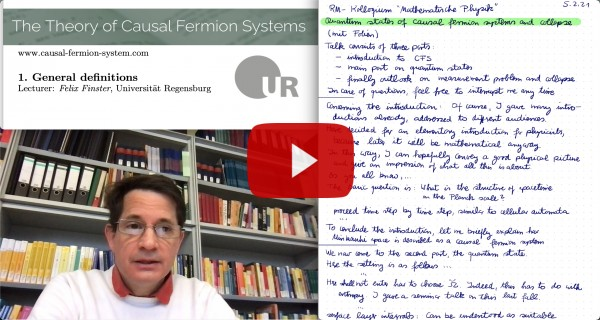# The Theory of Causal Fermion Systems

## Existence TheoryThe first paper where the existence of minimizers of the causal action was considered is [discrete05], where existence was proved for a system in discrete spacetime, in a formulation with indefinite inner product spaces.

For causal fermion systems, in order to make the causal action principle mathematically well-defined, one needs to specify the class of measures in which to vary $\rho$. To this end, on $\F$ we consider the topology induced by the operator norm $\|A\| := \sup \big\{ \|A u \|_\H \text{ with } \| u \|_\H = 1 \big\} \:.$ In this topology, the Lagrangian as well as the integrands in the trace and boundedness constraints are continuous. The $\sigma$-algebra generated by the open sets of $\F$ consists of the so-called Borel sets. The right prescription is to vary $\rho$ within the class of all regular Borel measures on $\F$ (a regular Borel measure is a measure on the Borel sets with the property that it is continuous under approximations by compact sets from inside and by open sets from outside).

It is proved in [continuum08] that minimizers exist if $\H$ is finite-dimensional and the total volume $\rho(\F)$ is finite. It is also shown in a homogeneous setting that minimizers exist in infinite volume, but in a formulation with indefinite inner product spaces and imposing a momentum cutoff.

The causal action principle is known to be ill-posed if the total volume $\rho(\F)$ is finite and the Hilbert space $\H$ is infinite-dimensional (see Exercises 1.1 and 1.2 in [cfs16]). But the causal action principle does make mathematical sense in the so-called infinite-dimensional setting when $\H$ is infinite-dimensional and the total volume $\rho(\F)$ is infinite. In this case, the volume constraint is implemented by demanding that all variations $(\rho(\tau))_{\tau \in (-\varepsilon, \varepsilon)}$ should for all $\tau, \tau’ \in (-\varepsilon, \varepsilon)$ satisfy the conditions $\big| \rho(\tau) – \rho(\tau’) \big|(\F) < \infty \qquad \text{and} \qquad \big( \rho(\tau) – \rho(\tau’) \big) (\F) = 0$ (where $|.|$ denotes the total variation of a measure). The existence theory in the infinite-dimensional setting is currently under investigation. As a preparatory step, in [noncompact20] the existence theory is developed for causal variational principles in the non-compact setting, typically for infinite total volume. Moreover, in [L21] some existence results in infinite dimensions are obtained.